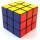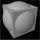Cubes

One cube is inscribed sphere and the other one described. Calculate difference of volumes of cubes, if the difference of surfaces in 257 mm2.

Result

V1-V2 =  512.6 mm3

Solution:Leave us a comment of example and its solution (i.e. if it is still somewhat unclear...):Math student
What do all those symbols mean? Is there a more simple format...that you could put this in?Petr
S - surface area of cube
V - volume of cube(s)To solve this example are needed these knowledge from mathematics:

Tip: Our volume units converter will help you with converion of volume units. Pythagorean theorem is the base for the right triangle calculator.

Next similar examples:

1. CubesCube, which consists of 27 small cubes with edge 4 dm has volume:
2. Cube in sphereThe sphere is inscribed cube with edge 8 cm. Find the radius of the sphere.
3. Cube from sphereWhat largest surface area (in cm2) can have a cube that was cut out of a sphere with radius 43 cm?
4. Sphere areaA cube with edge 1 m long is circumscribed sphere (vertices of the cube lies on the surface of a sphere). Determine the surface area of the sphere.
5. Cube volumeThe cube has a surface of 384 cm2. Calculate its volume.
6. Cube cornersFrom cube of edge 14 cm cut off all vertices so that each cutting plane intersects the edges 1 cm from the nearest vertice. How many edges will have this body?
7. HoleIn the center of the cube with edge 14 cm we will drill cylinder shape hole. Volume of the hole must be 27% of the cube. What drill diameter should be chosen?
8. Volume from surface areaWhat is the volume of the cube whose surface area is 96 cm2?
9. CuboidFind the cuboid that has the same surface area as the volume.
10. Sphere - partsCalculate the area of a spherical cap, which is part of an area with base radius ρ = 9 cm and a height v = 3.1 cm.
11. Fit ballWhat is the size of the surface of Gymball (FIT - ball) with a diameter of 65 cm?
12. GasholderThe gasholder has spherical shape with a diameter 20 m. How many m3 can hold in?
13. TetrahedronCalculate height and volume of a regular tetrahedron whose edge has a length 18 cm.
14. Triangular prismCalculate the surface area and volume of a triangular prism, base right triangle if a = 3 cm, b = 4 cm, c = 5 cm and height of prism h=12 cm.
15. Axial sectionAxial section of the cone is an equilateral triangle with area 208 dm2. Calculate the volume of the cone.
16. CalculationHow much is sum of square root of six and the square root of 225?
17. Theorem proveWe want to prove the sentence: If the natural number n is divisible by six, then n is divisible by three. From what assumption we started?